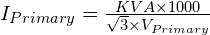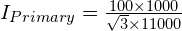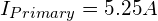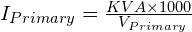# How to calculate Primary current of any transformer in just 2 steps!

How to calculate Primary current of any transformer in just 2 steps! 1024 576 Gaurav J

In this tutorial, I’ll explain 2 simple steps from which you can calculate primary current of any transformer. Transformer can be 1 phase or 3 phase. So, let’s get started.

## Step 1

##### Get the following details
• KVA/MVA rating of a transformer (For this example, assume KVA = 100)
• Primary Voltage of transformer (Assume 11kV)

## Step 2

##### Put the details obtained in Step 1 in the following formula

For 3 phase transformersLet’s put the values we assumed in Step 1 in above formula.For 1 phase transformersSo, these are the two simple steps you can use to calculate the primary current of any single phase or three phase transformer.

In case, you are interested is knowing the 3 simple steps to calculate the short circuit current of any transformer,###### Gaurav J

Electrical Engineer. Content Creator. Currently working with a High & Extra High Voltage Switchgear Industry.

All stories by: Gaurav J